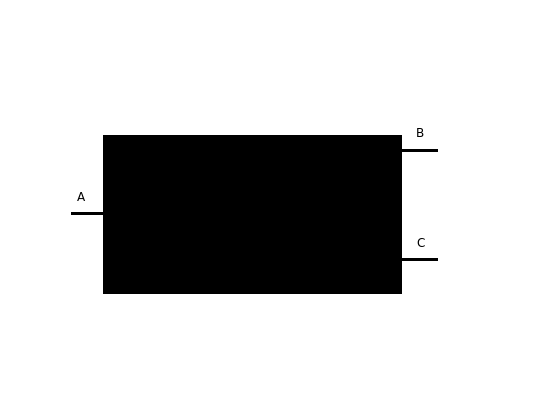# Black box

A circuit with 5 resistors of $1~\Omega$ is put into a black box, so that we don't know how the resistors are connected. If you measure the equivalent resistance between the points A and B, you get that it's $\frac{5}{3} \Omega$. The equivalent resistance between the points A and C is $\frac{8}{3}\Omega$. There are two possible equivalent resistances between B and C. One of those resistances is $1\Omega$. What is the possibility for the other resistance between the points B and C in $\Omega$?×

Problem Loading...

Note Loading...

Set Loading...